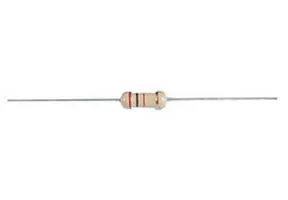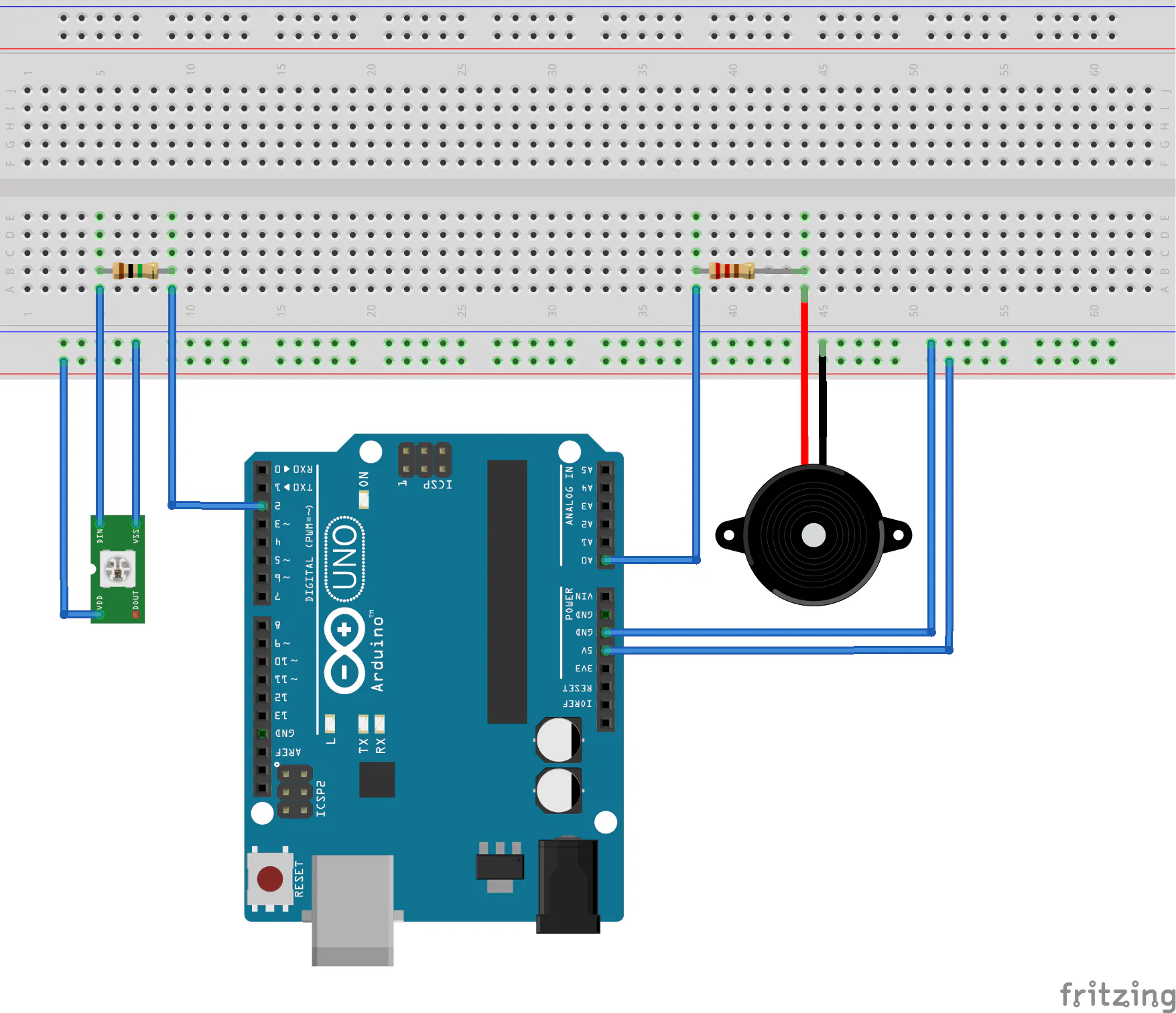# Bongo Hero: A Fun and Easy Arduino Game

Bongo Hero is inspired by Guitar Hero. You must tap bongos at the right time. Color changes and speed increases as you level up.

BeginnerShowcase (no instructions)2 hours5,881

## Things used in this project

### Hardware componentsArduino UNO & Genuino UNO
×1
 Adafruit NeoPixel LED Strip Starter Pack - 30 LED meter
×4
 SparkFun Piezo element
×4Resistor 220 ohm
×4
 SparkFun Resistor 1M ohm
×4

### Software apps and online servicesArduino IDE

## Schematics

### Bongo Hero schematics## Code

### Bongo Hero source code

Arduino
```#include <FastLED.h>

#define LED_TYPE             WS2811
#define NUM_STRIPS           4 // number of led strips, when changing this value, the setup function must be updated
#define BRIGHTNESS           64 // brightness of the leds
#define TILE_LENGTH          4 // number of leds per tile
#define COLOR_ORDER          GRB
#define MIN_INTERVAL         5 // the minimum number of leds switched off between two tiles
#define LEVEL_DURATION       5 // number of successful tap before level up
#define MIN_TILE_DELAY       50 // in milliseconds, minimum duration for a tile in a position
#define PIEZO_THRESHOLD      500
#define GAME_OVER_DELAY      1000 // in miliseconds, duration of the break when game is over
#define INIT_REFRESH_RATE    250 // in milliseconds, led strips refresh rate when game starts
#define NUM_LEDS_PER_STRIP   30 // number of leds per strip
#define MAX_TILES_PER_STRIP  3 // the maximum number of tiles present at the same time on a led strip
#define TILE_GENERATION_PROB 15 // probability to generate a new tile when possible the smaller it is, the more likely it is to generate one
#define LEVEL_SPEED_INCREASE 5 // in milliseconds, increase the speed of the led strip refresh rate when level

int score;
int currentDelay;
CRGB currentColor;
unsigned long lastTime;
int numTiles[NUM_STRIPS];
bool canCheck[NUM_STRIPS];
bool canCreate[NUM_STRIPS];
int currentIndex[NUM_STRIPS];
int tiles[NUM_STRIPS][MAX_TILES_PER_STRIP];
CRGB leds[NUM_STRIPS][NUM_LEDS_PER_STRIP];

void setup()
{
delay(3000); // power-up safety delay

InitGame();
randomSeed(37);
Serial.begin(9600);
FastLED.setBrightness(BRIGHTNESS);
}

void loop()
{
for(int i = 0; i < NUM_STRIPS; i++) {

// check if a bongo was hit
if(piezoValue > PIEZO_THRESHOLD) {
bool ok = false;
for(int j = 0; j < MAX_TILES_PER_STRIP; j++) {
if(tiles[i][j] < TILE_LENGTH*2 && tiles[i][j] != -1 && canCheck[i]) {
score++;
ok = true;
canCheck[i] = false;
FastLED.setBrightness(3*BRIGHTNESS);
break;
}
}

if(!ok && canCheck[i]) {
GameOver();
}
}
}

unsigned long currentTime = millis();

if(currentTime - lastTime > currentDelay) {
lastTime = currentTime;

// level up
if(score % LEVEL_DURATION == 0 && score > 0) {
currentDelay -= LEVEL_SPEED_INCREASE;
if(currentDelay < MIN_TILE_DELAY) currentDelay = MIN_TILE_DELAY; // maximum speed
currentColor = nextColor();
}

// one tile maximum will be created during each refresh
bool tileCreated = false;

// refresh display
for(int i = 0; i < NUM_STRIPS; i++) {
SwitchOffLEDs(leds[i]);

// Fill last tile
FillTile(TILE_LENGTH - 1, leds[i], CRGB::White);

if(numTiles[i] < MAX_TILES_PER_STRIP) {
// if there is enough space left on the strip
// we try to create a new tile
// 1/6 chance to create a new tile
if(canCreate[i] && random(0, TILE_GENERATION_PROB) == 0 && !tileCreated) {
numTiles[i]++;
tiles[i][currentIndex[i]] = NUM_LEDS_PER_STRIP - 1;
currentIndex[i]++;
tileCreated = true;
}
canCreate[i] = true;
}

// if tiles array is full,
// next tile will be created at index 0
if( currentIndex[i] == MAX_TILES_PER_STRIP ) {
currentIndex[i] = 0;
}

// Fill tiles on the strip
for(int j = 0; j < MAX_TILES_PER_STRIP; j++) {
if(tiles[i][j] == -1) continue;

if(!canCheck[i] && tiles[i][j] < TILE_LENGTH + MIN_INTERVAL && MIN_INTERVAL >= TILE_LENGTH) {
// We don't show a tile if it has already been taken in account by the player.
// This cannot be done this way if MIN_INTERVAL < TILE_LENGTH,
// in this case we'll have a fallback behaviour (we'll see the tile leaving the strip).
} else {
FillTile(tiles[i][j], leds[i], currentColor);
}
tiles[i][j]--;

// if tile exits the strip we decrement the number of tiles
if(tiles[i][j] == 0){
numTiles[i]--;
tiles[i][j] = -1;
if(score > 0) {
if(canCheck[i]) {
GameOver();
}
canCheck[i] = true;
}
}

// check if the tile is at the beginning of the strip
if(tiles[i][j] >= NUM_LEDS_PER_STRIP - TILE_LENGTH - MIN_INTERVAL) canCreate[i] = false;
}
}

FastLED.show();
FastLED.setBrightness(BRIGHTNESS);
}
}

void FillTile(int tile, CRGB leds[], CRGB color)
{
for(int i = tile; i > tile - TILE_LENGTH; i--) {
leds[i] = color;
if (i == 0) break;
}
}

void SwitchOffLEDs(CRGB leds[])
{
for(int i = 0; i < NUM_LEDS_PER_STRIP; i++) {
leds[i] = CRGB::Black;
}
}

void InitGame()
{
score = 0;
lastTime = millis();
currentColor = CRGB::Yellow;
currentDelay = INIT_REFRESH_RATE;

for(int i = 0; i < NUM_STRIPS; i++) {
numTiles[i] = 0;
canCheck[i] = true;
canCreate[i] = true;
currentIndex[i] = 0;

for(int j = 0; j < MAX_TILES_PER_STRIP; j++) {
tiles[i][j] = -1;
}
}
}

void GameOver()
{
for(int i = 0; i < NUM_STRIPS; i++) {
// Fill last tile in red
FillTile(TILE_LENGTH - 1, leds[i], CRGB::Red);
}
FastLED.show();
delay(GAME_OVER_DELAY);
InitGame();
}

CRGB nextColor() {
switch (score) {
case LEVEL_DURATION:
return CRGB::Blue;
case 2*LEVEL_DURATION:
return CRGB::Green;
case 3*LEVEL_DURATION:
return CRGB::Purple;
case 4*LEVEL_DURATION:
return CRGB::Orange;
case 5*LEVEL_DURATION:
return CRGB::Pink;
case 6*LEVEL_DURATION:
return CRGB::Salmon;
case 7*LEVEL_DURATION:
return CRGB::DeepSkyBlue;
case 8*LEVEL_DURATION:
return CRGB::ForestGreen;
default:
return CRGB::Yellow;
}
}
```

### Bongo Hero on Github

Github repository of the Bongo Hero project